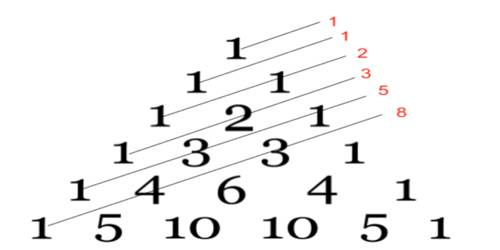# Fibonacci Sequence

Fibonacci Sequence

The Fibonacci sequence is a series of numbers where a number is found by adding up the two numbers before it starts with 0 and 1, the sequence goes 0,1,1,2,3,5,8,13,21,34, and so forth written, as a rule, the expression is Xn = Xn – 1 + Xn – 2;

• The 2 is found by adding the two numbers before it (1+1)
• The 3 is found by adding the two numbers before it (1+2),
• And the 5 is (2+3), and so on!

Fibonacci was known to be the most talented Western mathematician of the Middle Ages. Named after Fibonacci was also known as Leonardo of Pisa or Leonardo pisona, Fibonacci numbers were first introduced in his “Liber abaci” in 1202; The son of a Pisan merchant. Math was incredibly important to those in the trading industry, and his passion for numbers was cultivated in his youth. It is one of the most famous formulas in mathematics.

Knowledge of numbers is said to have first originated in the Hindu Arabic arithmetic system, which Fibonacci studied while growing up in North America. Prior to the publication of Liber Abaci.

The Latin-speaking world had yet to be introduced to the decimal number system. He wrote many books about geometry, commercial arithmetic, and irrational numbers. He also helped develop the concept of zero.